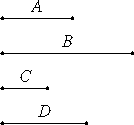# Proposition 24

If two numbers have to one another the ratio which a square number has to a square number, and the first is square, then the second is also a square.

Let the two numbers A and B have to one another the ratio which the square number C has to the square number D, and let A be square.I say that B is also square.

VIII.18

Since C and D are square, C and D are similar plane numbers. Therefore one mean proportional number falls between C and D.

And C is to D as A is to B, therefore one mean proportional number falls between A and B also. And A is square, therefore B is also square.

Therefore, if two numbers have to one another the ratio which a square number has to a square number, and the first is square, then the second is also a square.

Q.E.D.

## Guide

The proof of this proposition is straightforward.